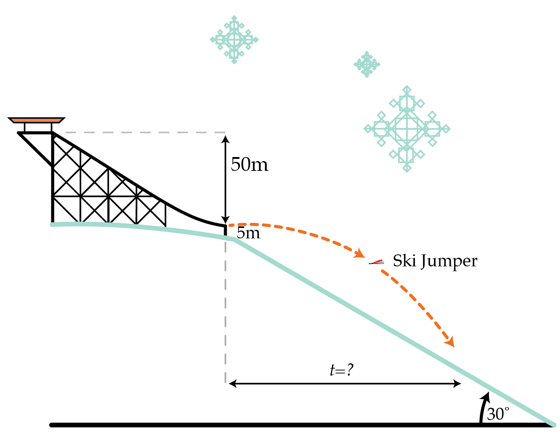# Ski jumping

A ski jumper launches from a ski jump that is oriented parallel to a hill. The jump has a vertical drop of 50 m and the coefficient of friction $\mu$ between the skier and the jump is 0.05. The launch point is 5 m above the hill and there is a small lip at the bottom of the jump so that the skier launches horizontally. How long in seconds is the skier in flight?Details and assumptions

• Neglect air resistance.
• The acceleration of gravity is $-9.8~m/s^2$.
• The hill slopes down at an angle of $\theta=30^o$ below the horizontal.
• The skier started from rest at the top of the jump.
×

Problem Loading...

Note Loading...

Set Loading...